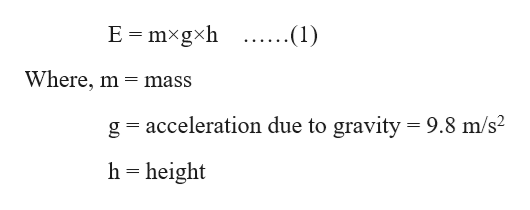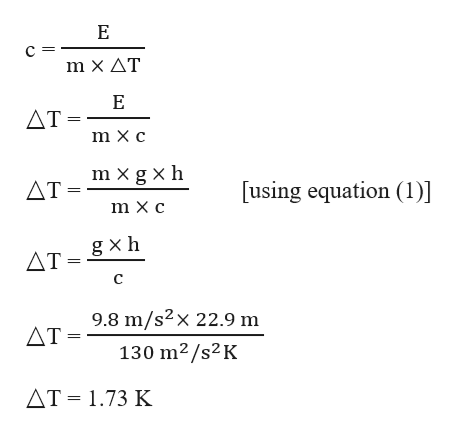# A bag filled with lead shots is dropped from a height of h = 22.9 m. The total mass of the bag is m = 620.0 g. What is the increase in the temperature of the lead shots, after the bag hits the ground? The specific heat of lead is c = 130 J/kgK.

Question
143 views

A bag filled with lead shots is dropped from a height of h = 22.9 m. The total mass of the bag is m = 620.0 g. What is the increase in the temperature of the lead shots, after the bag hits the ground? The specific heat of lead is c = 130 J/kgK.

check_circle

Step 1

Given,

Height, h = 22.9 m

Mass, m = 620.0 g

Specific heat, c = 130 J/kgK

We know that, 1 J = 1 kg m2/s2

⸫ Specific heat, c = 130 kg m2/s2kgK = 130 m2/s2K

Increase in temperature, △T = ?

Step 2

Energy (E) after the bag hits the ground can be given as:help_outlineImage TranscriptioncloseE mxgxh ....(1) Where, m mass g acceleration due to gravity = 9.8 m/s2 h height fullscreen
Step 3

The specific heat (c)...help_outlineImage TranscriptioncloseE с- m Χ ΔΤ E ΔΤ- m X c m x g xh ΔΤ- [using equation (1)] m X c g xh ΔΤΗ C 9.8 m/s2x 22.9 m ΔΤ- 130 m2/s2 K AT 1.73 K fullscreen

### Want to see the full answer?

See Solution

#### Want to see this answer and more?

Solutions are written by subject experts who are available 24/7. Questions are typically answered within 1 hour.*

See Solution
*Response times may vary by subject and question.
Tagged in

### Chemistry